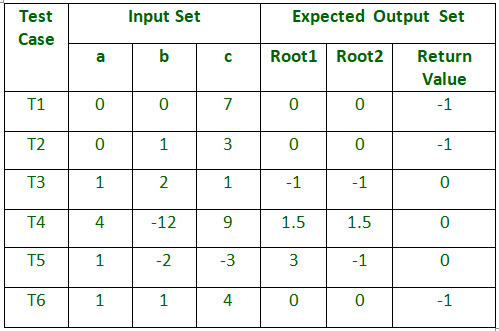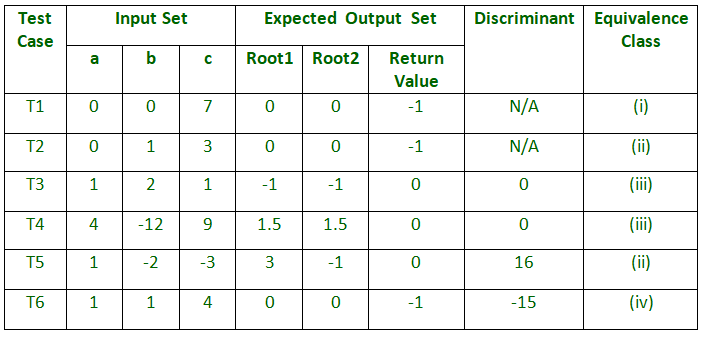Related Articles

# GATE | GATE CS 2011 | Question 47

• Last Updated : 14 Feb, 2018

The following is the comment written for a C function.

```
/* This function computes the roots of a quadratic equation
a.x^2 + b.x + c = . The function stores two real roots
in *root1 and *root2 and returns the status of validity
of roots. It handles four different kinds of cases.
(i) When coefficient a is zero irrespective of discriminant
(ii) When discreminant is positive
(iii) When discriminant is zero
(iv) When discriminant is negative.
Only in case (ii) and (iii) the stored roots are valid.
Otherwise 0 is stored in roots. The function returns
0 when the roots are valid and -1 otherwise.
The function also ensures root1 >= root2
int get_QuadRoots( float a, float b, float c,
float *root1, float *root2);
*/```

A software test engineer is assigned the job of doing black box testing. He comes up with the following test cases, many of which are redundant.Which one of the following option provide the set of non-redundant tests using equivalence class partitioning approach from input perspective for black box testing?
(A) T1,T2,T3,T6
(B) T1,T3,T4,T5
(C) T2,T4,T5,T6
(D) T2,T3,T4,T5

Explanation: